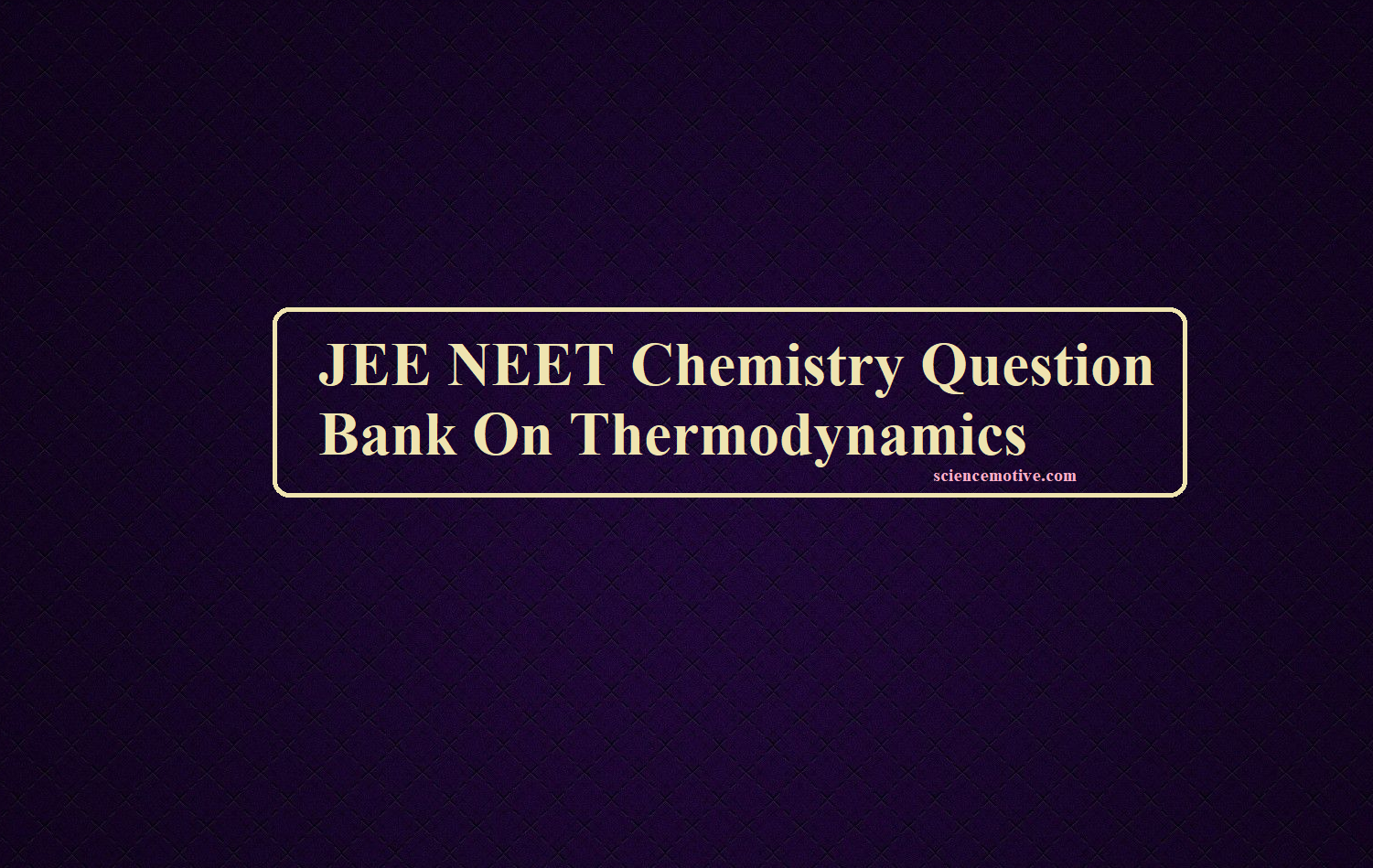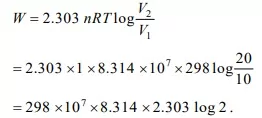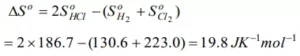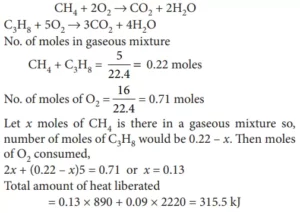# JEE NEET Chemistry Question Bank On Thermodynamics# JEE NEET Chemistry Question Bank On Thermodynamics

JEE NEET Chemistry Question Bank

1. Which of the following statement is false?
(a) Internal energy of an ideal gas depends only on the temperature.
(b) The relation between ∆U and ∆H is ∆H = ∆U + P∆V.
(c) Heat of a reaction at constant pressure is equal to HR – HP.
(d) Heats of reaction at constant pressure (∆H) and at constant volume (∆U) are related as ∆H – ∆U = ∆nRT.
Ans 1. (c) Heat of a reaction at constant pressure is equal to HR – HP.

2. The work done in ergs for the reversible expansion of one mole of an ideal gas from a volume of 10 litres to 20 litres at 25 0C is
(a) 2.303 × 298 × 0.082 log2                              (b) 298 × 107 × 8.31 × 2.303 log2
(c) 2.303 × 298 × 0.082 log0.5               (d) 8.31 × 107 × 298 – 2.303 log0.5        (e) 2.303× 298 × 2 log2
Ans 2. (b)3. The law of conservation of energy states that
(a) The internal energy of a system is constant                (b) The heat content of a system is constant
(c) Energy is neither created nor destroyed                      (d) There is an equivalence between energy and mass
Ans 3. (c) Energy is neither created nor destroyed

4. The enthalpies of the elements in their standard states are assumed to be
(a) Zero at 298 K           (b) Unit at 298 K           (c) Zero at all temperatures        (d) Zero at 273 K
Ans 4. a) The enthalpies of all elements in their standard state at 25 0C or 298K are zero

5. Heat of combustion ΔH for C(s), H2(g) and CH4(g) are – 94, – 68 and – 213 kcal/mol, then ΔH for
C(s) + 2H2(g) → CH4(g) is
(a) – 17 kcal                  (b) – 111 kcal                (c) – 170 kcal                (d) – 85 kcal
Ans 5. (a)
(i) C(s) + O2(g) → CO2(g); ΔHi = – 94 kcal/mole
(ii) 2H2(g) +O2(g) → 2H2O(l); ΔHii = – 68 × 2 kcal/mole
(iii) CH4(g) + 2O2(g) → CO2(g) + 2H2O(l); ΔHiii = – 213 kcal/mole
(iv) C(s) + 2H2(g) → CH4(g); ΔHiv = ?
By applying Hess’s law, we can compute ΔHiv + ΔHiv = ΔHi + ΔHii – ΔHiii
= (–94 – 68 × 2 + 213) kcal = –17 kcal

6. Change in enthalpy for reaction, 2H2O2(l) → 2H2O(l) + O2(g) if the heat of formation of H2O2(l) and H2O(l) are – 188 and – 286 kJ/mol respectively, is
(a) – 196 kJ/mol            (b) + 196 kJ/mol            (c) + 948 kJ/mol            (d) – 948 kJ/mole
Ans 6. (a)
ΔH°f = ΣH°f(products) – ΣH°f(reactants)
For the given reaction, 2H2O2(l) → 2H2O(l) + O2(g) DH°f
= 2 ×Δ°f(H2O) – 2 × ΔH°f(H2O2) = 2 × –286 kJ mol–1 – 2 × (–188) kJ mol–1 = – 196 kJ mol–1

JEE NEET Chemistry Question Bank

7. The heat of combustion of carbon to CO2 is – 393.5 kJ/mol. The heat released upon formation of 35.2 g of CO2 from carbon and oxygen gas is
(a) + 315 kJ                  (b) – 630 kJ                  (c) – 3.15 kJ                  (d) – 315 kJ
Ans 7. (d)
C(s) + O2(g) → CO2(g), ΔH = – 393.5 kJ/mol
Amount of heat released on formation of 44 g CO2 = – 393.5 kJ
Amount of heat released on formation of 35.2 g of CO2 = – 393.5/44 × 35 2 = – 314.8 ≈ – 315 kJ

8. When 5 litres of a gas mixture of methane and propane is perfectly combusted at 0°C and 1 atmosphere, 16 litres of oxygen at the same temperature and pressure is consumed. The amount of heat released from this combustion in kJ (ΔHcomb(CH4) = 890 kJ mol–1, ΔHcomb(C3H8) = 2220 kJ mol–1) is
(a) 38                           (b) 317                         (c) 477                          (d) 32
Ans 8. (b)
CH4 + 2O2 → CO2 + 2H2O
C3H8 + 5O2 → 3CO2 + 4H2O
No. of moles in gaseous mixture CH4 + C3H8 = 5/22.4 = 0.22 moles
No. of moles of O2 = 16/22.4 = 0.71 moles
Let x moles of CH4 be there in a gaseous mixture so, the number of moles of C3H8 would be 0.22 – x.
Then moles of O2 consumed, 2x + (0.22 – x)5 = 0.71 or x = 0.13
Total amount of heat liberated = 0.13 × 890 + 0.09 × 2220 = 315.5 kJ

9. An irreversible process occurring isothermally in an isolated system leads to
(a) Zero entropy                                                                      (b) An increase in the total entropy of the system
(c) A decrease in the total entropy of the system             (d) None of these
Ans 9.  (b)
The entropy of the system increases as the process occur irreversibly and isothermally in an isolated system.

10. The entropy values (in JK-1 mol-1) of H2(g) = 130.6, Cl2(g) = 223.0 and HCl (g) = 186.7 at 298 K and 1 atm pressure. Then entropy change for the reaction
H2(g) + Cl2(g) ® 2HCl(g) is
(a) + 540.3                                (b) + 727.3                    (c) – 166.9                    (d) + 19.8
Ans 10. (d)JEE NEET Chemistry Question Bank

11. Which one of the following processes is non-spontaneous
(a) Dissolution of CuSO4 in water          (b) Reaction between H2 and O2 to form water
(c) Water flowing downhill                       (d) Flow of electric current from low potential to high potential
Ans 11. (d) The flow of electric current from low potential to high potential

12. The entropy of crystalline substances at absolute zero going by the third law of thermodynamics should be taken as
(a) 100                          (b) 50                           (c) Zero                        (d) Different for different substances
Ans 12. (c) It is the third law of thermodynamics.

13. For which of the following reactions the change in enthalpies is equal to the change in internal energy?
(a) H2 + I2 → 2HI                                              (b) PCl5 → PCl3 + Cl2
(d) 2H2O2 → 2H2O + O2                                    (d) C + O2 → CO2
Ans 13. (a) H2 + I2 → 2HI

14. For a reaction, 2NH3(g) → N2(g) + 3H2(g) which of the following statements is correct?
(a) ∆H > ∆U                 (b) ∆H < ∆U                 (c) ∆H = ∆U                 (d) ∆H = 0
Ans 14. (a) ∆H > ∆U

15. The heat of combustion of carbon to CO2 is –393.5 kJ/mol. The heat released upon formation of 35.2 g of CO2 from carbon and oxygen gas is
(a) + 315 kJ                  (b) – 630 kJ                  (c) – 3.15 kJ                  (d) – 315 kJ
Ans 15. C(s) + O2(g) → CO2(g), ∆H = – 393.5 kJ/mol
Amount of heat released on formation of 44 g CO2 = 393.5 kJ/mol
Amount of heat released on formation of 35.2 g of CO2 = 393.5/44 × 35.2 = 314.8 ≈ 315 kJ

16. When 5 litres of a gas mixture of methane and propane is perfectly combusted at 0°C and 1 atmosphere, 16 litres of oxygen at the same temperature and pressure is consumed. The amount of heat released from this combustion in kJ (∆Hcomb (CH4) = 890 kJ mol–1, ∆Hcomb. (C3H8) = 2220 kJ mol–1) is
(a) 38                           (b) 317                         (c) 477                          (d) 32
Ans 16. (b) 31717. Bond dissociation enthalpy of H2, Cl2 and HCl are 434, 242 and 431 kJ mol–1 respectively. Enthalpy of formation of HCl is
(a) – 93 kJ mol–1                        (b) 245 kJ mol1                        (c) 93 kJ mol–1              (d) – 245 kJ mol–1
Ans 17. (a)
H2 + Cl2 → 2HCl
∆Hreaction = Σ(B.E)reactants – Σ(B.E)products
= [(B.E)H – H + (B.E)Cl – Cl] – [2B.E(H – Cl)]
= 434 + 242 – (431) × 2
∆Hreaction = – 186 kJ
Heat of formation is the amount of heat absorbed or evolved when one mole of substance is directly obtained from its constituent elements.
Hence, enthalpy of formation of HCl = – 186/2 = – 93 kJ mol–1

18. The entropy of crystalline substances at absolute zero going by the third law of thermodynamics should be taken as
(a) 100                          (b) 50                           (c) Zero                        (d) Different for different substances
Ans 18. (c) Zero

19. In which state, the matter has the highest entropy
(a) Solid                       (b) Liquid                     (c) Gas                         (d) Equal in all
Ans 19. (c) The entropy of gases is the highest.

20. A reaction occurs spontaneously if
(a) TΔS < ΔH and both ΔH and ΔS are +ve                     (b) TΔS > ΔH and ΔH is +ve and ΔS is –ve
(c) TΔS > ΔH and both ΔH and ΔS are +ve                     (d) TΔS = ΔH and both ΔH and ΔS are +ve
Ans 20. (c)
ΔG = ΔH – TΔS ΔG = –ve for spontaneous reaction. When ΔS = +ve, ΔH = +ve and TΔS > ΔH
⇒ ΔG = –ve

JEE NEET Chemistry Question Bank